# Nikodým boundedness theorem

(diff) ← Older revision | Latest revision (diff) | Newer revision → (diff)

A theorem [a5], [a4], saying that a family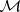of countably additive signed measures(cf. Measure) defined on a-algebra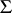and pointwise bounded, i.e. for each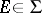there exists a number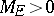such that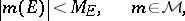is uniformly bounded, i.e. there exists a number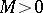such that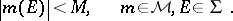As is well-known, the Nikodým boundedness theorem for measures fails in general for algebras of sets. But there are uniform boundedness theorems in which the initial boundedness conditions are imposed on certain subfamilies of a given-algebra; those subfamilies need not be-algebras. The following definitions are useful [a2], [a7], [a8]:

SCP) An algebrahas the sequential completeness property if each disjoint sequence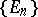fromhas a subsequencewhose union is inSIP) An algebrahas the subsequential interpolation property if for each subsequence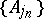of each disjoint sequence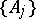fromthere are a subsequenceand a set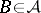such thatand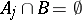for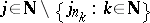.

The Nikodým boundedness theorem holds on algebras with SCP) and SIP).

A famous theorem of J. Dieudonné [a3] states that for compact metric spaces the pointwise boundedness of a family of regular Borel measures on open sets implies its uniform boundedness on all Borel sets. There are further generalizations of this theorem [a6].

How to Cite This Entry:
Nikodým boundedness theorem. Encyclopedia of Mathematics. URL: http://encyclopediaofmath.org/index.php?title=Nikod%C3%BDm_boundedness_theorem&oldid=15213
This article was adapted from an original article by E. Pap (originator), which appeared in Encyclopedia of Mathematics - ISBN 1402006098. See original article